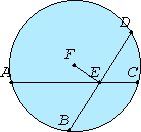# Proposition 4

If in a circle two straight lines which do not pass through the center cut one another, then they do not bisect one another.

Let ABCD be a circle, and in it let the two straight lines AC and BD, which do not pass through the center, cut one another at E.I say that they do not bisect one another.

III.1

For, if so, let them bisect one another, so that AE equals EC, and BE equals ED. Take the center F of the circle ABCD. Join FE.

III.3

Then, since a straight line FE passing through the center bisects a straight line AC not passing through the center, it also cuts it at right angles, therefore the angle FEA is right.

III.3

Again, since a straight line FE bisects a straight line BD, it also cuts it at right angles. Therefore the angle FEB is right.

But the angle FEA was also proved right, therefore the angle FEA equals the angle FEB, the less equals the greater, which is impossible.

Therefore AC and BD do not bisect one another.

Therefore if in a circle two straight lines which do not pass through the center cut one another, then they do not bisect one another.

Q.E.D.

## Guide

This proposition is not used in the rest of the Elements. The contrapositive of this statement is more positive: if two straight lines in a circle bisect each other, then they meet at the center.

This proposition is not used in the rest of the Elements.